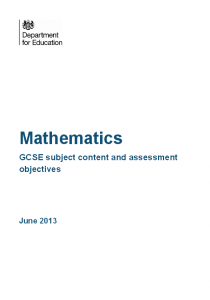# Summary of proposed changes to GCSE Mathematics

• Grades 8 to 1 (not corresponding to A* to G – aim is to even out the distribution more)
• Still tiered; still “overlapping” tiers but degree of overlap may well change
• Assessment by terminal examination only;  minimum assessment time 3.5 hours
• No “SPAG” marks in Mathematics
• Still Nov resit – but considering Year 12 and older only to prevent early/repeated entry
• Detailed feedback in each area unlikely

### Changes to ContentMore formulae need to be recalled including:

• Volume/surface area of a cone and sphere
• Volume of a prism
• Area of a trapezium
• Sine/Cosine Rules and Area of a non-right angled triangle
• Velocity and acceleration

• Algebra
• Kinematics (three “suvat” equations are given)
• Error intervals using inequality notation
• Function notation including composition of two functions/inverses; construct and apply functions to problems
• Graph of y=kx for positive integer k
• Identify and interpret gradients, roots, intercepts, turning points of quadratic functions graphically; deduce roots algebraically and turning points by completing the square
• Quadratic inequalities, including solution sets in set notation
• Nth term of a quadratic sequence
• More graphs including exponential, piece-wise; problem solving e.g. kinematic problems, financial graphs; calculating area under graphs
• Ratio, proportion and rates of change
• Rates of change, represented graphically and algebraically.
• Geometry
• Identify, describe and construct shapes transformed by stretch parallel to an axis; identify invariant points and lines of each transformation
• Apply circle theorems in geometric proofs (as well as proving the theorems themselves)
• Construct geometric arguments and proofs using vectors
• Describe changes and invariance after a combination of transformations
• Probability
• Conditional probability; using Venn diagrams and the formulae P(A or B) = P(A) + P(B) – P(A and B), P(A and B) = P(A given B)P(A)
• Calculating lines of best fit
• Expected outcomes compared to long-run averages
• Statistics
• Interpolate and extrapolate trends

Thanks to Ian Davies, the ARK Network Lead for Mathematics, for compiling this information.

## 3 Replies to “Summary of proposed changes to GCSE Mathematics”

1.srcav says:

Good summary, how do you feel about it? I think the new topics are a good step forward, but was hoping more set theory would be brought in, as well as group theory and an introduction to calculus. I don’t see the benefit of changing grades from letters to numbers and feel it will cause confusion.

2.gcsemaths says:

It is about time that harder topics were introduced to the syllabus